# Momentum Trading: Tutorial, Strategies & Review

Updated: 25 June 2020

Momentum trading is a strategy that involves taking advantage of price volatility and strong moves in prices by buying in an uptrend and selling when that trend loses momentum.• How momentum is calculated
• Two momentum trading strategies based on longer-term price charts

Momentum trading is a financial market strategy approach that capitalises on big and fast moves in the underlying price of a security.  Traders will look to buy securities when they are rising and sell them when they are falling.

In essence, momentum is the speed at which market values are changing in an asset.

Momentum readings can be further classified into two distinct categories: Absolute Momentum and Relative Momentum.

Absolute Momentum
Market behaviour in the price of an asset is based on its relationship to past performances using historical time sequences.

Relative momentum
Market behaviours in the prices of individual securities are compared with one another (showing relative strength that differentiates between strong-performing assets and weak-performing securities).

## How Is Momentum Calculated?

First, traders must understand what the word “momentum” actually means in practical terms.  For these purposes, a straightforward definition of the concept can be interpreted as follows:

Momentum = Underlying Trend Strength

Let’s consider market price activity using three different examples:

Positive Momentum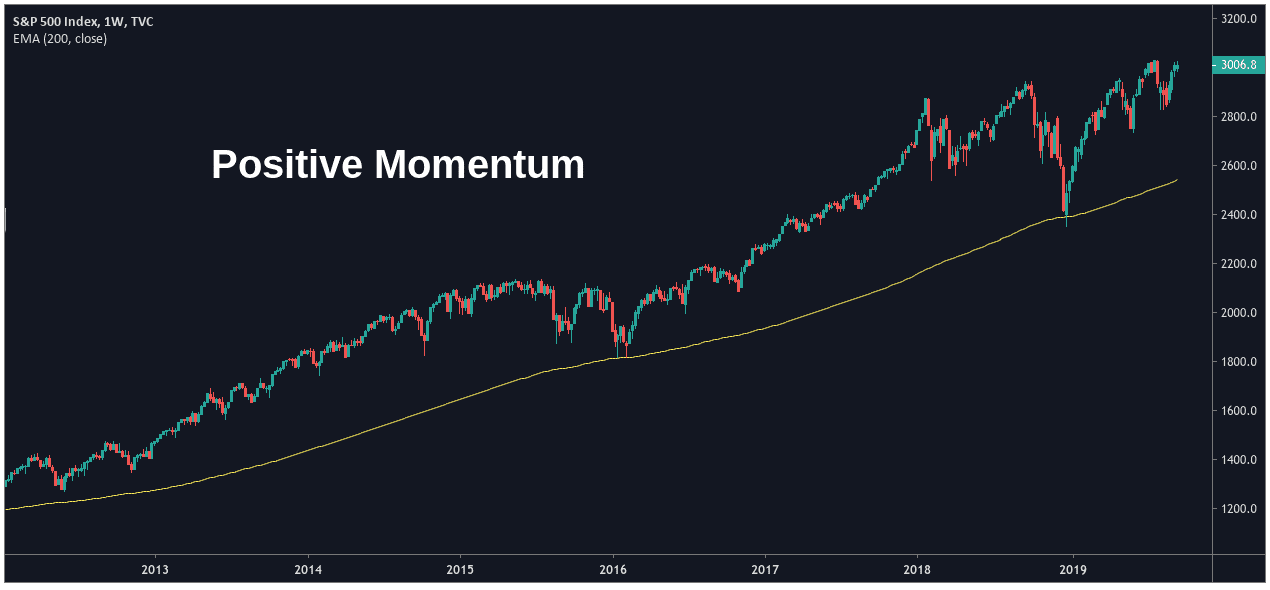In our first example, we can see a market showing positive price momentum. In this case, the asset is consistently holding above its 200-period exponential moving average (EMA) while its trend continues moving higher.

Negative Momentum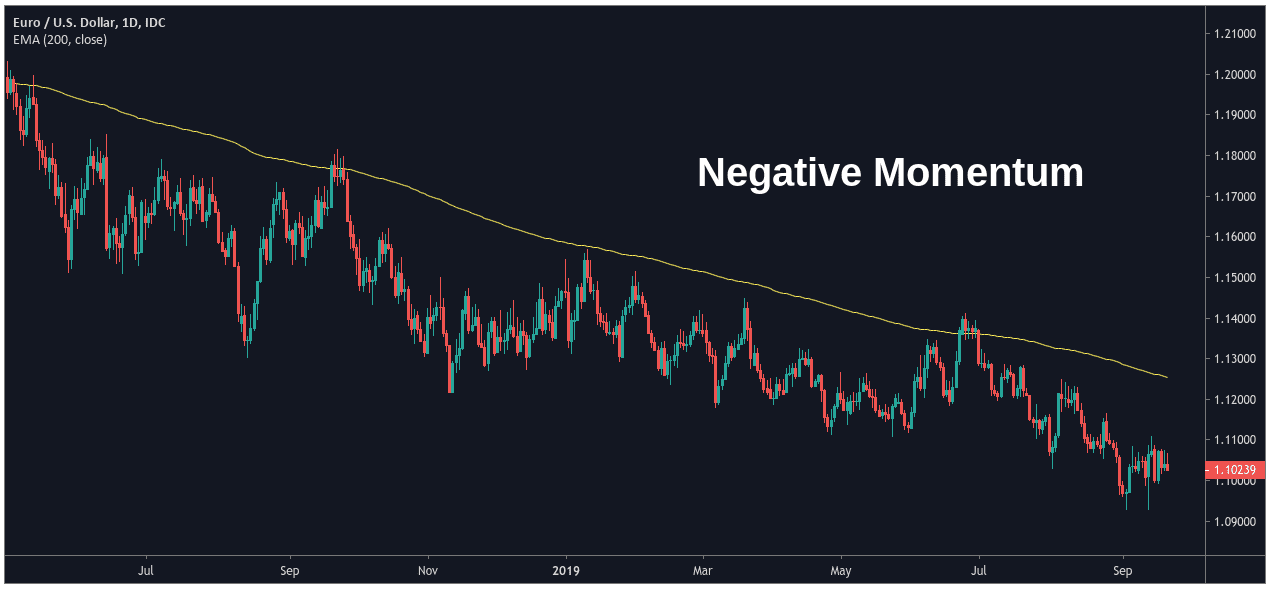In our second example, we can see a market showing negative momentum.  In this case, the asset consistently holds below the 200-period EMA while it continues moving lower.

No Directional Momentum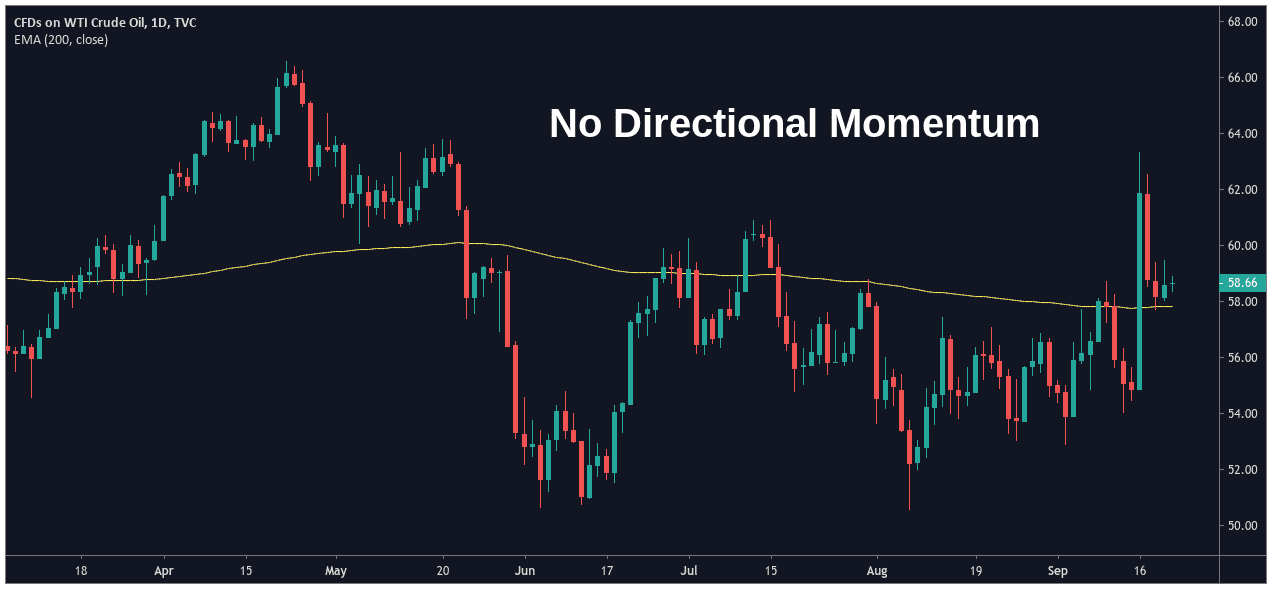In our third example, we can see a market with no clear directional momentum.  In this case, the asset price consistently oscillates above and below its 200-period EMA.  Prices are trading in an erratic fashion (while moving sideways) and there is no significant trend dictating the value of the asset.

There are many indicators that can be used to judge the momentum of an asset. Some of the best momentum indicators are:

With these indicators in mind, let’s look at some of the strategies you can use for momentum trading.

### Strategy 1

What is needed:

• 200 period moving average
• Daily chart

The Average Directional Index (ADX) is a popular trading tool that is used to determine the trend momentum of an asset.

As the ADX level rises, it indicates a strengthening trend. In other words, the trend might be bullish or it might be bearish  — all we know is that the underlying trend is gaining strength.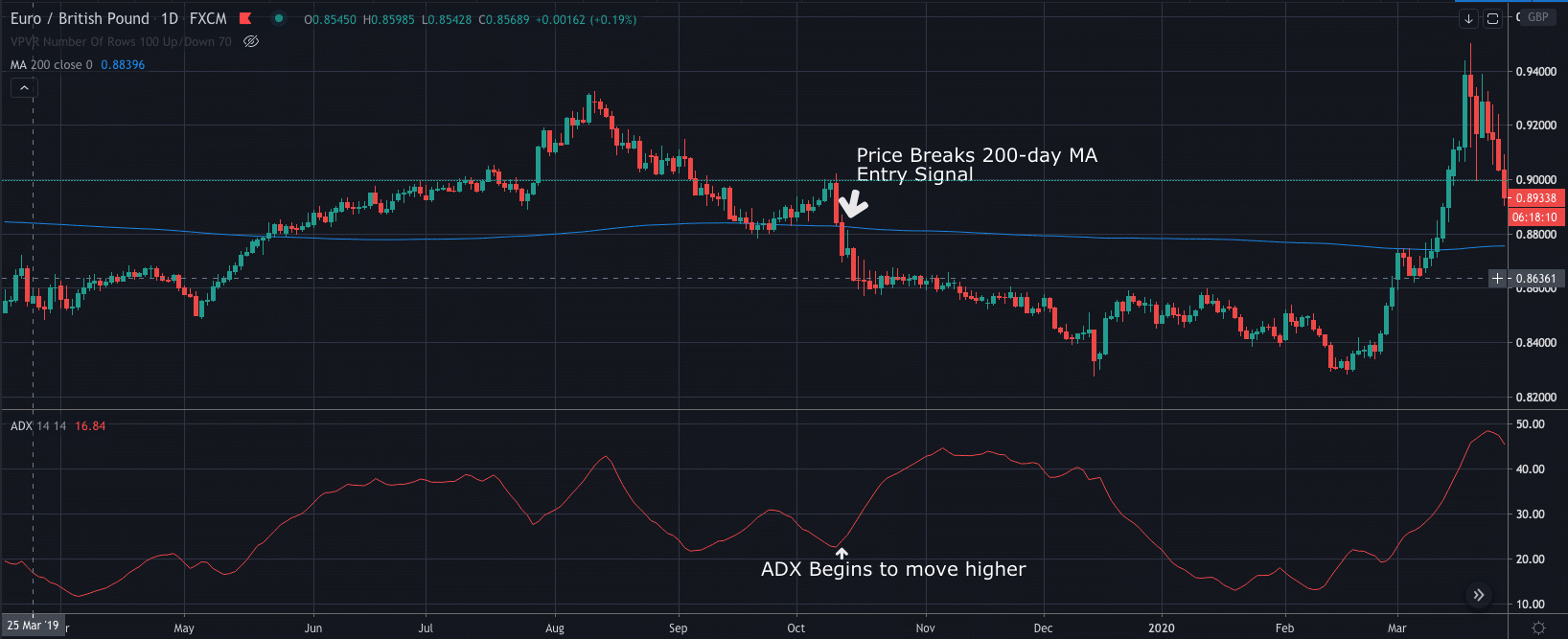To the left of the price chart, we can see that the EURGBP price begins moving higher.

As the ADX value rises towards 100, we can see that the underlying trend is also strengthening.

In this example, the ADX strengthens as the EURGBP price moves above the 200-period moving average. This essentially gives us two independent pieces of evidence that suggest strength is building in the underlying price trend.

How to enter a position:

• Wait for the ADX to trend higher
• Wait until price breaks the 200-day moving average

Once you have entered your long position your stop-loss should be placed under the most recent swing in price, while your target is 2x that. Giving you a 2:1 profit ratio.

### Strategy 2

Another strategy traders can use is to spot divergences in price action.

What you will need for this strategy is:

• RSI
• Four-hour chart

Divergence is when price trends in one direction, but the indicator (in this case the RSI) starts to trend in the opposite direction.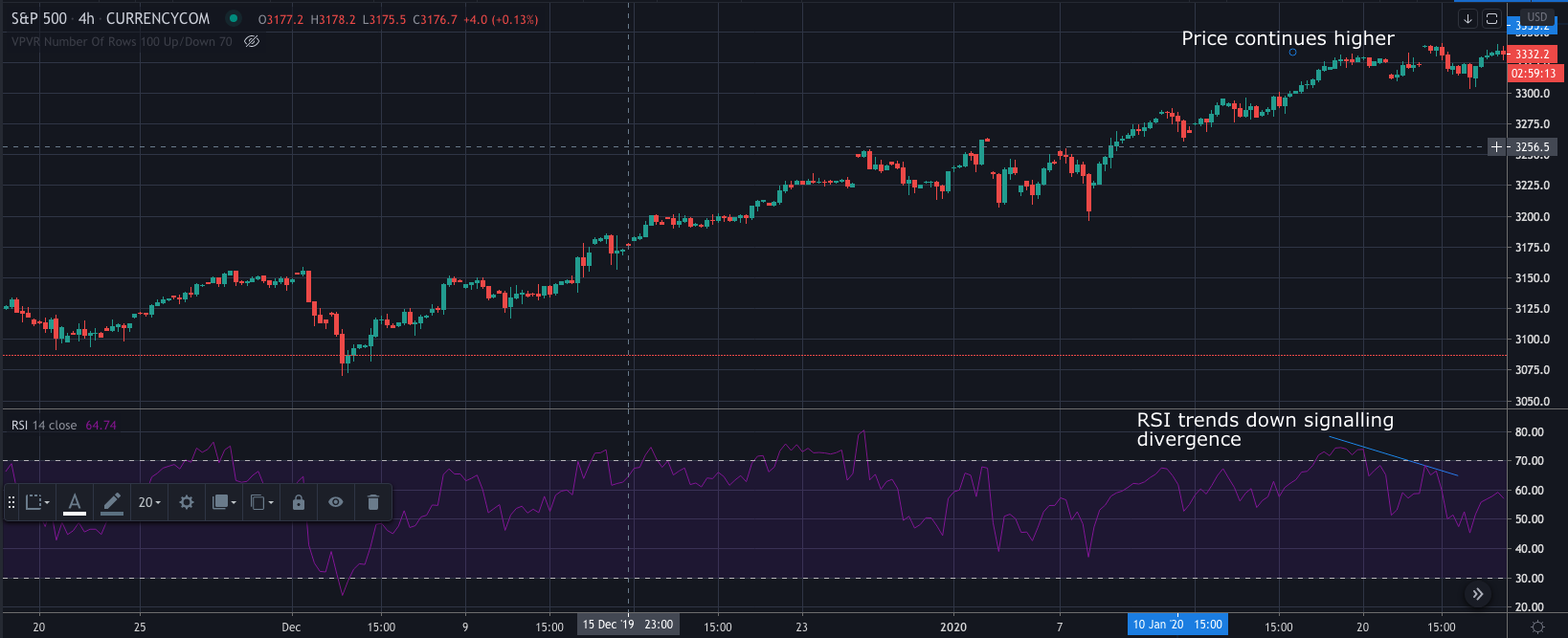In the example shown above, we can see that the S&P 500 price is rallying to reach record highs. However, we can also see that the RSI indicator is making lower highs, signalling divergence.

So, to enter a position we would look for:

• Price trending in one direction
• RSI Trends lower
• Enter in the direction of the RSI (in the above case this would be a sell position)

Similar to the first strategy the stop-loss would go above or below the recent swing in price, while the take-profit target will be the next key level, that is at least 2x our stop.

There are some fantastic aspects to momentum trading that can help you to make quick profits. However, there are also negative aspects as well.

So let’s take a look at both sides:

• Big profits in a short period – fast moves as momentum builds.
• Great for day trading – As profits can be taken quickly, you can take short term trades (although it can also be used on longer timeframes as shown in the strategies above).
• Take advantage of the volatility – you can capitalise when markets surge in one direction.